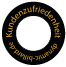96%
Excellent
9.59/10

## Company

Zip codes in Germany have 5 numerical digits! (e.g. 12345)
Zip codes in Bahrain have 3 or 4 numerical digits! (e.g. 123 or 1234)
Zip codes in Bulgaria have 4 numerical digits! (e.g. 1234)
Zip codes in Denmark have 4 numerical digits! (e.g. 1234)
Zip codes in France have 5 numerical digits! (e.g. 12345)
Zip codes in Italy have 5 numerical digits! (e.g. 12345)
Zip codes in Croatia have 5 numerical digits! (e.g. 12345)
Zip codes in Liechtenstein have 4 numerical digits! (e.g. 1234)
Zip codes in Luxembourg have 4 numerical digits! (e.g. 1234)
Zip codes in Holland have 4 numerical digits, a blank and 2 alphanumerical digits! (e.g. 1234 AB)
Zip codes in Austria have 4 numerical digits! (e.g. 1234)
Zip codes in Poland have 2 numerical digits, a hyphen and 3 numerical digits! (e.g. 12-123)
Zip codes in Portugal have 4 numerical digits, a hyphen and 3 alphanumerical digits! (e.g. 1234-123)
Postleitzahlen in Rumänien sind 6-stellig und bestehen aus Ziffern! 123456
Zip codes in Sweden have 3 numerical digits, a blank and 2 numerical digits! (e.g. 123 12)
Zip codes in Switzerland have 4 numerical digits! (e.g. 1234)
Zip codes in Slovakia have 3 numerical digits, a blank and 2 numerical digits! (e.g. 123 12)
Zip codes in Czech Republic have 3 numerical digits, a blank and 2 numerical digits! (e.g. 123 12)
Zip codes in Turkey have 5 numerical digits! (e.g. 12345)
Zip codes in the USA can be 5 digits or 5 digits with a hyphen followed by 4 more digits (eg 12345 or 12345-1234)
The zip code is not in a propper form for Great Britain!

Zip codes in Germany have 5 numerical digits! (e.g. 12345)
Zip codes in Bahrain have 3 or 4 numerical digits! (e.g. 123 or 1234)
Zip codes in Bulgaria have 4 numerical digits! (e.g. 1234)
Zip codes in Denmark have 4 numerical digits! (e.g. 1234)
Zip codes in France have 5 numerical digits! (e.g. 12345)
Zip codes in Italy have 5 numerical digits! (e.g. 12345)
Zip codes in Croatia have 5 numerical digits! (e.g. 12345)
Zip codes in Liechtenstein have 4 numerical digits! (e.g. 1234)
Zip codes in Luxembourg have 4 numerical digits! (e.g. 1234)
Zip codes in Holland have 4 numerical digits, a blank and 2 alphanumerical digits! (e.g. 1234 AB)
Zip codes in Austria have 4 numerical digits! (e.g. 1234)
Zip codes in Poland have 2 numerical digits, a hyphen and 3 numerical digits! (e.g. 12-123)
Zip codes in Portugal have 4 numerical digits, a hyphen and 3 alphanumerical digits! (e.g. 1234-123)
Postleitzahlen in Rumänien sind 6-stellig und bestehen aus Ziffern! 123456
Zip codes in Sweden have 3 numerical digits, a blank and 2 numerical digits! (e.g. 123 12)
Zip codes in Switzerland have 4 numerical digits! (e.g. 1234)
Zip codes in Slovakia have 3 numerical digits, a blank and 2 numerical digits! (e.g. 123 12)
Zip codes in Czech Republic have 3 numerical digits, a blank and 2 numerical digits! (e.g. 123 12)
Zip codes in Turkey have 5 numerical digits! (e.g. 12345)
Zip codes in the USA can be 5 digits or 5 digits with a hyphen followed by 4 more digits (eg 12345 or 12345-1234)
The zip code is not in a propper form for Great Britain!
The fields marked with * are required

## My benefits

• Express Shopping
• Save your user data and settings
• Overview of your orders incl. shipping informations